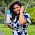## Tuesday, 10 July 2012

### Simple Button Debouncing Code in Verilog

So you have made a counter and after programming it onto your board you realize that every button press increments the counter by 30 or 40 units. This problem is knows as bouncing and to overcome this a debouncing circuit is needed to compensate for the mechanical button bounces.

The push buttons and switches on the FPGA boards are mechanical devices and tend to bounce multiple times when pressed. And since the code related to the button is usually placed in the always @ (posedge clock) block, every bounce of the button is picked up and processed. It has been found that the bounces last around 20 ms after which it stabilizes. The debouner circuit should be able to filter out these bounces and only pick up the stabilized state of the button.

Since it is known that the bounces last around 20 ms the first thing the code should have is a timer, a timer that will outlast the 20 ms of instability. I will use a 10 ms counter and after every 10 ms will check the state of the button, if it has stabilized it should process the button press, if not the value from the button is to be ignored.

To better understand the bouncing problem of the button consider the following input waveform from the button:

The code for the debouncing circuit is given below. I have used FSM to code it and it has been commented in detail to explain each step. I hope it is clear enough. I have tested this and it works in simulation and on the basys2 board. I will be using this code for every project I do from now on.

```module debouncing(
input clock,
input reset,
input button,
output reg out
);

localparam N = 19;        //for a 10ms tick

reg [N-1:0]count;
wire tick;

// the counter that will generate the tick.

always @ (posedge clock or posedge reset)
begin
if(reset)
count <= 0;
else
count <= count + 1;
end

assign tick = &count;        //AND every bit of count with itself. Tick will only go high when all 19 bits of count are 1, i.e. after 10ms

// now for the debouncing FSM

localparam[2:0]                     //defining the various states to be used
zero = 3'b000,
high1 = 3'b001,
high2 = 3'b010,
high3 = 3'b011,
one = 3'b100,
low1 = 3'b101,
low2 = 3'b110,
low3 = 3'b111;

reg [2:0]state_reg;
reg [2:0]state_next;

always @ (posedge clock or posedge reset)
begin
if (reset)
state_reg <= zero;
else
state_reg <= state_next;
end

always @ (*)
begin
state_next <= state_reg;  // to make the current state the default state
out <= 1'b0;                    // default output low

case(state_reg)
zero:
if (button)                    //if button is detected go to next state high1
state_next <= high1;
high1:
if (~button)                //while here if button goes back to zero then input is not yet stable and go back to state zero
state_next <= zero;
else if (tick)                //but if button remains high even after 10 ms, go to next state high2.
state_next <= high2;
high2:
if (~button)                //while here if button goes back to zero then input is not yet stable and go back to state zero
state_next <= zero;
else if (tick)                //else if after 20ms (10ms + 10ms) button is still high go to high3
state_next <= high3;
high3:
if (~button)                //while here if button goes back to zero then input is not yet stable and go back to state zero
state_next <= zero;
else if (tick)                //and finally even after 30 ms input stays high then it is stable enough to be considered a valid input, go to state one
state_next <= one;

one:                                //debouncing eliminated make output high, now here I'll check for bouncing when button is released
begin
out <= 1'b1;
if (~button)        //if button appears to be released go to next state low1
state_next <=  low1;
end
low1:
if (button)                //while here if button goes back to high then input is not yet stable and go back to state one
state_next <= one;
else if (tick)            //else if after 10ms it is still high go to next state low2
state_next <= low2;
low2:
if (button)                //while here if button goes back to high then input is not yet stable and go back to state one
state_next <= one;
else if (tick)            //else if after 20ms it is still high go to next state low3
state_next <= low3;
low3:
if (button)                //while here if button goes back to high then input is not yet stable and go back to state one
state_next <= one;
else if (tick)            //after 30 ms if button is low it has actually been released and bouncing eliminated, go back to zero state to wait for next input.
state_next <= zero;
default state_next <= zero;

endcase
end

endmodule```

#### 1 comment:

1.could you please explain me the relation between the LOCAL PARAMETER (N) and NO. OF BITS IN THE COUNT..!